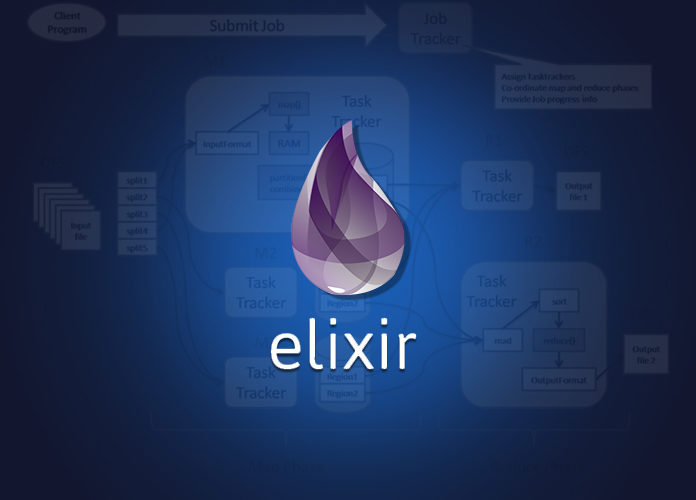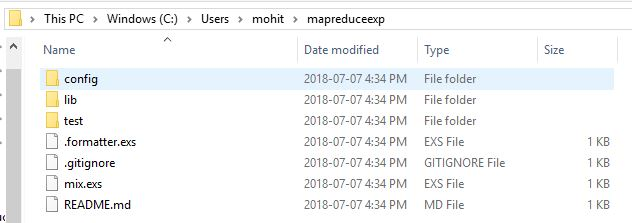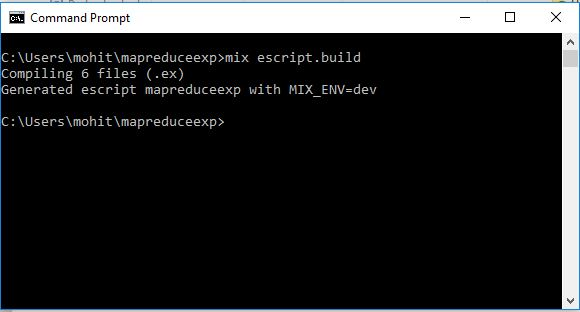# Implementing MapReduce function in Elixir

0
1222MapReduce is a well known pattern which is widely used in Big Data for the analysis of a concurrent data set. In the world of functional programming, Map and Reduce are two higher order functions. Map function takes a list and lambda or an anonymous function as its arguments and applies the provided function to each element present in the supplied list, and returns a new list as output given by the lambda function. Reduce function works similar to Map function in terms of accepting the same arguments i.e. a list and lambda function along with an additional argument in Elixir i.e. an accumulator, and returns an accumulated value instead of list. In this article, we are going to learn the concurrency and MapReduce as powerful Elixir’s features along with a suitable example that demonstrate MapReduce feature.

### Map Reduce example – A word count application

Before, we start working on Elixir’s Map reduce example it is expected that your system should have Elixir installed. Elixir installation steps and kick start example was discussed in the last article. Let’s create a new project for building word count application by using the following syntax.

 1 mix new mapreduceexp –module MapReduce

The above syntax will create your Mix project with project directory mapreduceexp [C:\Users\xyz\mapreduceexp].

```Microsoft Windows [Version 10.0.17134.112]

C:\Users\xyz>mix new mapreduceexp --module MapReduce
* creating .formatter.exs
* creating .gitignore
* creating mix.exs
* creating config
* creating config/config.exs
* creating lib
* creating lib/mapreduceexp.ex
* creating test
* creating test/test_helper.exs
* creating test/mapreduceexp_test.exs

Your Mix project was created successfully.
You can use "mix" to compile it, test it, and more:

cd mapreduceexp
mix test

Run "mix help" for more commands.```In the Next step, you need to navigate into your mapreduceexp directory and edit mix.exs with the line inside def project do [] as shown below.

```defmodule MapReduce.MixProject do
use Mix.Project

def project do
[
app: :mapreduceexp,
version: "0.1.0",
elixir: "~> 1.6",
escript: [main_module: MapReduce],
start_permanent: Mix.env() == :prod,
deps: deps()
]
end

# Run "mix help compile.app" to learn about applications.
def application do
[
extra_applications: [:logger]
]
end

# Run "mix help deps" to learn about dependencies.
defp deps do
[
# {:dep_from_hexpm, "~> 0.3.0"},
# {:dep_from_git, git: "https://github.com/elixir-lang/my_dep.git", tag: "0.1.0"},
]
end
end```

## MapReduce function Flow

MapReduce in Elixir can be demonstrated as a pipeline through the data flows in 5 steps. These 5 steps correspond to 5 modules which will be written in Elixir programming language.
Input Reader: The step 1 is the creation of input reader in Elixir that reads data as input and split the data into a format that could be easily read by our Map process and simultaneously launches Map processes. Code implementation is given below.

`lib\input_reader_module.ex`
```defmodule InputReaderModule do
require Mapper

{:ok, body}      -> Enum.each(Regex.split( ~r/\r|\n|\r\n/, String.trim(body)), fn line -> spawn(fn -> Mapper.map(line, partition) end) end)
{:error, reason} -> IO.puts :stderr, "File Error: #{reason}"
end
end
end```

Map process: The Map process reads the formatted data from input reader and executes a function on each piece of supplied data to provide a key value pair to a Partition or Compare process as output. Code implementation is given below.

`lib\mapper_module.ex`
```defmodule MapperModule do
def map(line, partition) do
send(partition, {:process_put, self()})
Enum.each(String.split(line, " "), fn key -> send(partition, {:value_put, key}) end)
end
end```

Partition or Compare process: The Partition process accepts the accumulated key value pairs supplied by the Map process in order to compare these pairs and issues Reduce processes for each unique key. Code implementation is given below.

`lib\partition_module.ex`
```defmodule PartitionModule do
require ReducerModule
require OutputWriterModule

end

defp loop(processes, values) do
mailbox_length = elem(Process.info(self(), :message_queue_len), 1)
if (mailbox_length == 0), do: (
mapper_check(processes, Keyword.delete(Keyword.delete(values, String.to_atom(~s(\s))), String.to_atom("")))
)
{:process_put, caller} ->
loop([caller | processes], values)
{:value_put, key} ->
loop(processes, [{String.to_atom(key), 1} | values])
error -> IO.puts :stderr, "PartitionModule Error: #{error}"
end
end

defp mapper_check(processes, values) do
check = Enum.filter(processes, fn process -> Process.alive?(process) == true end)
uniques = Enum.uniq(Keyword.keys(values))
if (length(check) == 0 && length(uniques) != 0), do: (
Enum.each(uniques, fn unique -> spawn(fn -> ReducerModule.reduce(Keyword.to_list(Keyword.take(values, [unique])), output_writer) end) end)
)
end
end```
• Reduce process: The Reduce function executes a function on each value which adds up all the values for the given key in order to emit these values to the Output Writer. Code implementation is given below.
`lib\reducer_module.ex`
```defmodule ReducerModule do
def reduce(tuples, output_writer) do
send(output_writer, {:process_put, self()})
case tuples do
[] ->  IO.puts :stderr, "Empty List"
tuples ->
send(output_writer, {:value_put, "#{elem(hd(tuples), 0)} #{Enum.reduce(tuples, 0, fn ({_k, v}, total) -> v + total end)}"})
end
end
end```
• Output writer: The output writer accepts the process data from Reduce process in the pipeline and yields the processed data in the desired format. Code implementation is given below.
`lib\output_writer_module.ex`
```defmodule OutputWriterModule do
end

defp loop(processes, values) do
mailbox_length = elem(Process.info(self(), :message_queue_len), 1)
if (mailbox_length == 0), do: (
reducer_check(processes, values)
)
{:process_put, caller} ->
loop([caller | processes], values)
{:value_put, value} ->
loop(processes, [value | values])
end
end

defp reducer_check(processes, values) do
check = Enum.filter(processes, fn process -> Process.alive?(process) == true end)
if (length(check) == 0 && length(processes) != 0), do: (
{:ok, file} = File.open(Path.join("test", "output.txt"), [:write])
for value <- values do
IO.puts value
IO.write(file, value <> ~s(\n))
end
File.close(file)
Process.exit(self(), :kill)
)
end
end```
• Main function to call modules and functions: In this step, you need to navigate into C:\Users\xyz\mapreduceexp\lib project directory and open mapreduceexp. ex file. Here first of you need to add the following imports to modules [InputReaderModule, PartitionModule] as shown below. Next, define the main function [def main(args) do], pipeline function when no file is given, pipeline function with options arguments, and parse_args function. All of these functions are given below as a part of code implementation.
```defmodule MapReduce do
require PartitionModule

def main(args) do
args |> parse_args |> pipeline
end

defp pipeline([]) do
IO.puts "No file given"
end

defp pipeline(options) do
forever()
end

defp forever do
forever()
end

defp parse_args(args) do
{options, _, _} = OptionParser.parse(args,
switches: [file: :string]
)
options
end
end```

Building and Executing Application

At this point our app development demonstrating MapReduce has completed, next we need to issue command [mix escript.build ] in order to build the application. The application will generate an app file named mapreduceexp as shown below.Next, we can execute this app file after issuing the command [mix run mapreduceexp –file=text\inputtext.txt]to observe the Map Reduce output for word count.

Conclusion